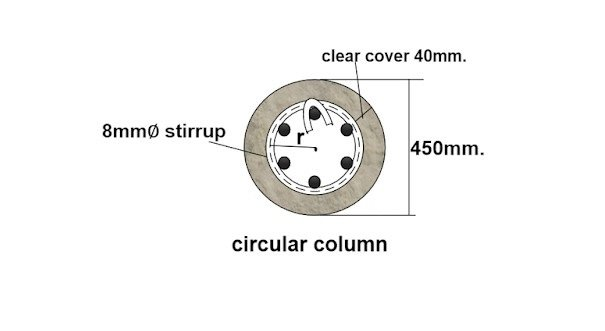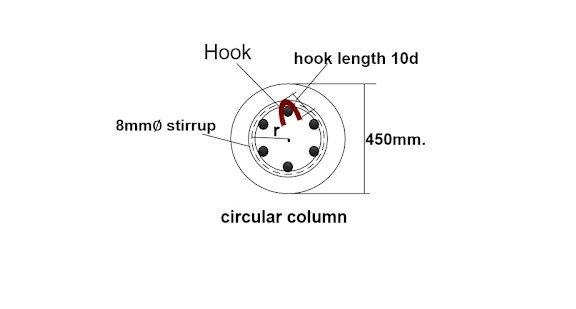Breaking News
Home / Civil Engineering / Bar Bending Schedule / How To Calculate The Cutting Length Of Circular Stirrup

# How To Calculate The Cutting Length Of Circular Stirrup

### How To Calculate The Cutting Length Of Circular Stirrup

Now, let us calculate the cutting length of the circular stirrup for the below-given drawing.### Given data

Diameter of circular column D = 450mm

Clear cover = 40mm.

The diameter of stirrup d = 8mm.

### The cutting length of the circular stirrup

= [2πr + ( 2nos. × hook length ) – ( 2nos. × hook bend. ) ]

= [ 2πr +( 2nos. × 10d ) – ( 2nos. × 2d )### Here,

d = diameter of the stirrup.

r = radius of the centerline of the circular stirrup ( as shown in the drawing).

First, we will calculate the value of r from the given data.

r = [ (1/2 × diameter of column ) –  clear cover – ( 1/2 × diameter of stirrup bar )]

r =  [ (1/2 × 450mm ) – 40mm – ( 1/2 × 8mm ) ]

r = [ 225mm – 40mm – 4mm ]

### r = 181mm.

1. Calculation Method For Find Out The Overlapping Length Of Beam, Column And Slab

### The cutting length of the circular stirrup

= [ ( 2 × π × 181mm ) + ( 2nos × 10d ) – ( 2nos. × 2d ) ]

= [ ( 2 × 3.142 × 181mm ) + ( 2nos. × 10 × 8mm ) – ( 2nos. × 2 × 8mm. )]

= [ 1137.4 mm + 160mm – 32mm ]

## Other Post

### THANKS.